Project tutorial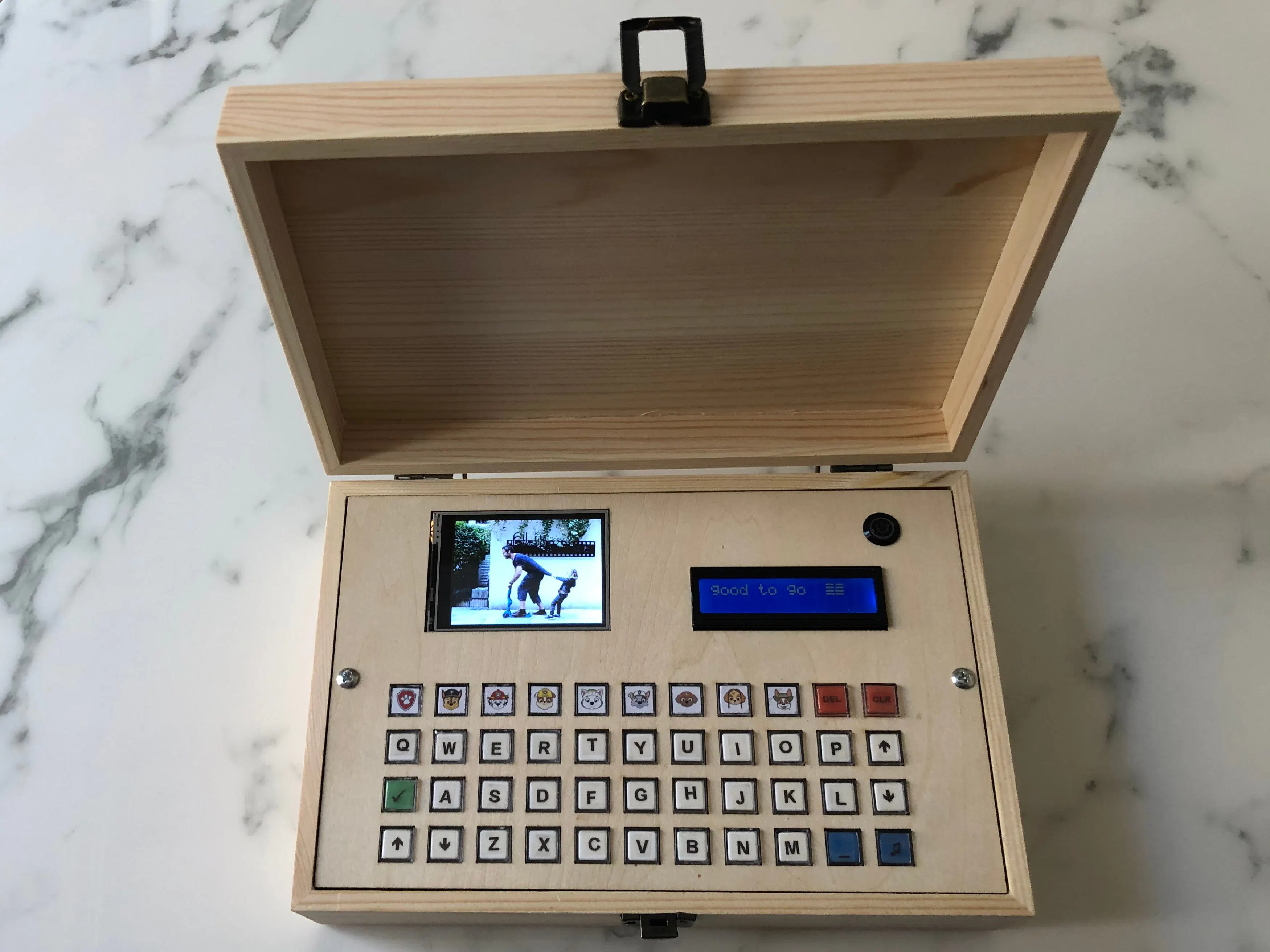# My Daughter's Laptop © CC BY-NC-ND

It's a programable "laptop." Hardware that allows me to program different activities in a nice box.

• 5,762 views
• 23 respects

## Components and supplies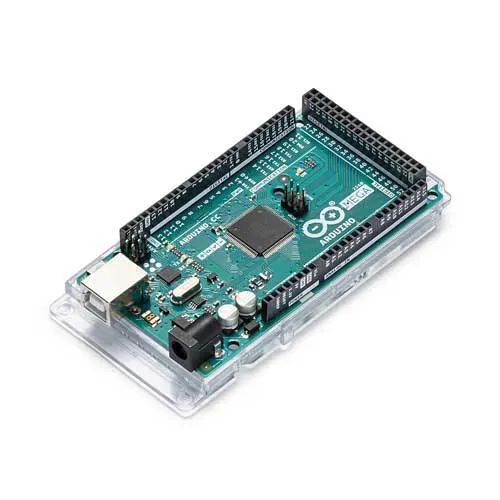Arduino Mega 2560
×1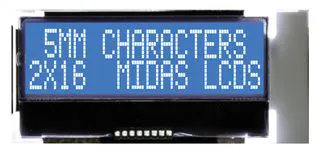Alphanumeric LCD, 16 x 2
×1
 ILI9341
×1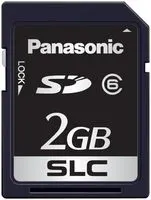Flash Memory Card, SD Card
×2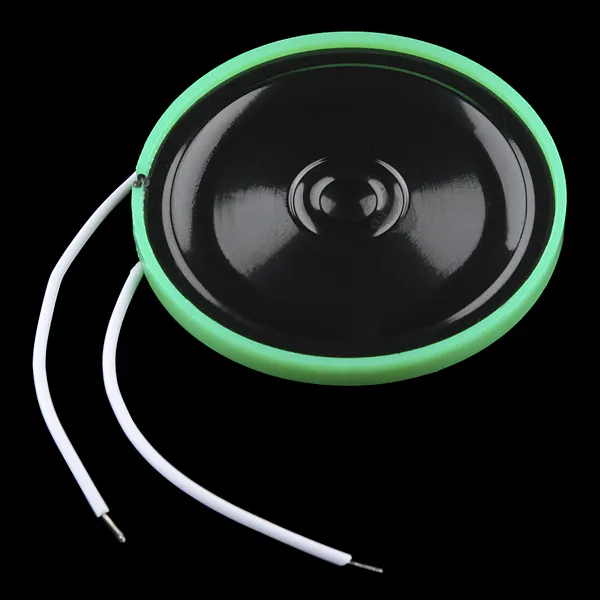Speaker: 0.25W, 8 ohms I use a 3W one
×1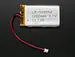Li-Ion Battery 1000mAh
×1
 DFPlayer - A Mini MP3 Player
×1
 3.7V 4.2V Charger & 5V 6V 9V 12V Discharger Board DC DC Converter Boost Module for UPS mobile power 18650 lithium battery
×1
 DC 5-12V NE555 Switch Module Relay Shield Timer 0-150 Second Adjustable L2KD
×1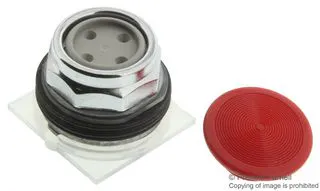Switch Actuator, Head for spring return push-button
×1

This is a long project, I will divide it into small bits so people can take pieces and set up their own. I also recommend seeing my previous projects to understand how I got here, especially the "tutorial for ILI9341 (part 1, 2, 3, and 4), as it uses some modified libraries that I would not explain here.

I dived the project into (power supply, MP3, LCD's, keyboard, and software)

The software is an on-going project, I would be adding different programs my daughter gets different interests.

Keyboard:

I used 2 keypads 4x4 and one 3x4 to make a 40 key keypad this brand makes a keypad that allows me to have that many keys with only 3 pins

THE LCD 16 x2

This part of the project displays words and whatever you type on the keyboard

PIN3 controls the brightness of the screen, this level is control from the keypad

LCD TFT SD CARD

This one is the messiest part of the whole project, but if you prepare the jumper wires in advance it makes a big difference at the very least consider preparing the wires for (MISO, MOSI, and CLK)

The SD Card is prepared with Images to show all of them are BMP (320 x 240) RGB

I use also the TFT Capabilities, for now is only showing one new picture when you touch the screen, but I wanted all prewired because come of the next program will include the touch-screen

MP3 DFR0299

This is a prepared MP3 Module that plays the files in order, you find all the details at dfrobot.com, so the big idea is to get all the sound bites you need, and then process them to have the same volume and then save them all numbered 0000.mp3 and so.

This module has many other options but I decided only to use those basic ones for this project.

The power supply.

All this part of the project can be just skipped if you are nor interested in making it work with batteries, I wanted to create a toy for my daughter and I wanted to be able to work with its own battery and not to need to always be connected.

First I have a wire with a USB female at the box from the female there is a wire direct to the USB entrance in the Arduino Mega that allows to program the box without having to open it but also splits to the battery charger

The charger I use is this one that allows me to connect any battery plus it suplies regulated output to the Arduino

Then it goes to a timer switch. This timer switch in conjunction with a push switch allows to keep the project going for 30 minutes a t a time so it will shut off after 30 minutes of being turn on, On the future I plan to connect the switch also to one of the Arduino Pins to turn off after 10 min of inactivity instead of 30 minutes after turning on ( I will post the diagram and the software adjustments to it)

The battery is just a 3, 7 lithium battery.

## Code

##### The code for the spelling game Arduino
This game shows a picture on the ILI9341. On the keyboard, there are 10 drawings.
After pressing one of those keys a Picture of the animal appears on the screen, the name is written on the LCS 16x2, and a track with the sound plays on the mp3. Then after you type it on the keyboard if the name is correct it sounds music to celebrate .
```//LCD
#include <ILI9341_kbv.h> // This library has been modified
ILI9341_kbv my_lcd;
#define vali0 0

//SD CARD
#include <SD.h>
#define TFT_SD 47  //SC_CS
Sd2Card card;
SdVolume volume;
SdFile root;

//MP3 Mini
#include "DFRobotDFPlayerMini.h"
//#include "Arduino.h"
#include "SoftwareSerial.h"
SoftwareSerial mySoftwareSerial(7,6); // RX, TX
DFRobotDFPlayerMini myDFPlayer;

//LCD 2 lines
#include <LiquidCrystal_I2C.h>// LCD  Library and variables
LiquidCrystal_I2C lcd(0x27,16,2);
int lcdLED = 3; // brignes of the LCD
int bright; // To store the screen brightness

//TFT Touch Library
#include <LCDWIKI_TOUCH.h>
LCDWIKI_TOUCH my_tft(44,52,50,51,46); //tcs,tclk (sharing with LCD),tdout (MISO) ,tdin (MOSI) ,tirq

//Folder on the SD card to keep the pictures
String FOLDERROOT = ""; // I have no folder because all the pictures are on the root of the SD
uint8_t max_img = 40;
uint8_t image_counter = 0;
unsigned long auxtimer, colortimer;

int analogPin0 = A0; // Or any pin you are using
int analogPin1 = A1; // Or any pin you are using
int analogPin2 = A2; // Or any pin you are using

//Aux Global variables
String song;
String dog;
String mydog;

void setup()
{
Serial.begin(9600);
//Initiate LCD big screen
my_lcd.begin();
my_lcd.setRotation(3);// Landscape
my_lcd.fillScreen(TFT_PINK);// set color iitial screen
//Initiate the Touch capabilities
SD.begin(TFT_SD);
my_tft.TP_Init(1,240,320);  //Define the values for my_lcd ( rotation,With,Height)

// initialize the lcd
Serial.print("\nInitializing LCD...");
bright =70;// brignes of the LCD
analogWrite(lcdLED, bright);// brignes of the LCD
lcd.init();
lcd.backlight();
lcd.setCursor(0,0);

//Inicialize mp3
Serial.print("\nInitializing MP3...");
mySoftwareSerial.begin(9600);
if (!myDFPlayer.begin(mySoftwareSerial)) //Use softwareSerial to communicate with mp3.
{
lcd.println("Unable to begin:");
// while(true); //wait until fixed
}
myDFPlayer.setTimeOut(500); //Set serial communictaion time out 500ms
myDFPlayer.volume(25);  //Set volume value (0~30).
myDFPlayer.EQ(DFPLAYER_EQ_NORMAL);
myDFPlayer.outputDevice(DFPLAYER_DEVICE_SD);

//All good

//Fist picture
Serial.print("\nInitializing BMP...");
String filename ="pp_1.bmp";
int len = 30;
char pepito[len];
filename.toCharArray(pepito, len);
bmpDraw(pepito , 0, 0);
lcd.init();
lcd.backlight();
lcd.setCursor(0,0);
myDFPlayer.play(28);
lcd.println("good to go  ");

}

// Makes an Array out of a BMP
void bmp (String filename){
int len = 30;
char pepito[len];
filename.toCharArray(pepito, len);
bmpDraw(pepito , 0, 0);}

String PAW (String dog)//PRINT THE NAME OF THE DOG
{
lcd.init();
lcd.setCursor(0,0);
lcd.print(dog);
MP3(dog);
delay(50);
mydog =""; //Zero mydog variable
return dog; // return the name of the dog to the main program
}

void TYPE( String key, String dog) // print the keyboar imput and compares to the dog name
{
lcd.setCursor(0,1);
lcd.print(mydog);
lcd.print(key);
if (key == "DEL") //delete last character of the string mydog
{
int mydoglenght = mydog.length();  // Check the lenght of the string mydog
mydoglenght =mydoglenght-1;
mydog.remove(mydoglenght, 1);
lcd.setCursor(0,1);
lcd.print(mydog);
lcd.print("     " );
}
else{ // Add character to the string mydog
mydog= mydog+key;
}
delay(500);
if  (mydog == dog)
{
lcd.print(" GREAT" ); // Positive message
myDFPlayer.play(35);
pinMode(9, HIGH);
delay(500);
}

}

void MP3 (String mp3Command)
{

if (mp3Command == "volumeup")  {myDFPlayer.volumeUp();}
if (mp3Command == "volumedown"){myDFPlayer.volumeDown();}
if (mp3Command == "pause")     {myDFPlayer.pause(); }
if (mp3Command == "STOP")      {myDFPlayer.stop(); }
if (mp3Command == "previous")  {myDFPlayer.previous(); }
if (mp3Command == "next")      {myDFPlayer.next();}
if (mp3Command == "play")      {myDFPlayer.next(); lcd.print("play");}

// Specific song tracks with the voice of the cartacters
if (mp3Command == "PAW PATROL") {myDFPlayer.play(22);}
if (mp3Command ==  "CHASE")     {myDFPlayer.play(4);}
if (mp3Command ==  "MARSHALL")  {myDFPlayer.play(13);}
if (mp3Command ==  "RUBBLE")    {myDFPlayer.play(27);}
if (mp3Command ==  "EVEREST")   {myDFPlayer.play(14);}
if (mp3Command ==  "ROCKY")     {myDFPlayer.play(10);}
if (mp3Command ==  "ZUMA")      {myDFPlayer.play(14);}
if (mp3Command ==  "SKYE")      {myDFPlayer.play(30);}
if (mp3Command ==  "TRACKER")   {myDFPlayer.play(14);}
if (mp3Command ==  "THEME")     {myDFPlayer.play(35);}

delay(100);
}

void show (String key) //Other functions on the keyboard
{

//Clear Screen
if (key == "CLR")
{
lcd.init();
lcd.setCursor(0,0);
mydog =""; //Zero mydog variable
}
//BRIGHTNES UP  Screen
if (key == "UP")
{
bright =bright +10;
analogWrite(lcdLED, bright);// brignes of the LCD
delay(200);
}
//BRIGHTNES DOWN  Screen
if (key == "DOWN") //Clear Screen
{
bright =bright -10;
analogWrite(lcdLED, bright);// brignes of the LCD
delay(200);
}
}

void loop(){

int val0 = 0;  // an auxiliar variable to store  the value
int val1 = 0;  // an auxiliar variable to store  the value
int val2 = 0;  // an auxiliar variable to store  the value

char key= ("");

val0 = analogRead (analogPin0); //4x4 kepay 2
if (val0 >= 1020 and val0 <= 1024){dog=PAW("PAW PATROL");bmp("pp_1.bmp");};
if (val0 >= 925 and val0 <= 935){dog=PAW("CHASE");bmp("pp_8.bmp");};
if (val0 >= 845 and val0 <= 855){dog=PAW("MARSHALL");bmp("pp_9.bmp");};
if (val0 >= 785 and val0 <= 795){dog=PAW("RUBBLE");bmp("pp3.bmp");};
if (val0 >= 675 and val0 <= 685){TYPE("Q" ,dog);};
if (val0 >= 630 and val0 <= 640){TYPE("W" ,dog);};
if (val0 >= 595 and val0 <= 605){TYPE("E" ,dog);};
if (val0 >= 560 and val0 <= 570){TYPE("R" ,dog);};
if (val0 >= 500 and val0 <= 510){key = ("OK");myDFPlayer.play(14);delay(500);};
if (val0 >= 480 and val0 <= 490){TYPE("A" ,dog);};
if (val0 >= 450 and val0 <= 460){TYPE("S" ,dog);};
if (val0 >= 435 and val0 <= 445){TYPE("D" ,dog);};
if (val0 >= 400 and val0 <= 410){MP3("volumeup");};
if (val0 >= 320 and val0 <= 330){MP3("volumedown");};
if (val0 >= 265 and val0 <= 275){TYPE("Z" ,dog);};
if (val0 >= 233 and val0 <= 243){TYPE("X" ,dog);};

val1 = analogRead (analogPin1); //4x4 keypad 1
if (val1 >= 1020 and val1 <= 1024){dog=PAW("EVEREST");bmp("pp_10.bmp");};
if (val1 >= 925 and val1 <= 935){dog=PAW("ROCKY");bmp("pp_22.bmp");};
if (val1 >= 845 and val1 <= 855){dog=PAW("ZUMA");bmp("pp_6.bmp");};
if (val1 >= 785 and val1 <= 795){dog=PAW("SKYE");bmp("pp_55.bmp");};
if (val1 >= 675 and val1 <= 685){TYPE("T" ,dog);};
if (val1 >= 630 and val1 <= 640){TYPE("Y" ,dog);};
if (val1 >= 595 and val1 <= 605){TYPE("U" ,dog);};
if (val1 >= 560 and val1 <= 570){TYPE("I" ,dog);};
if (val1 >= 500 and val1 <= 510){TYPE("F" ,dog);};
if (val1 >= 480 and val1 <= 490){TYPE("G" ,dog);};
if (val1 >= 450 and val1 <= 460){TYPE("H" ,dog);};
if (val1 >= 435 and val1 <= 445){TYPE("J" ,dog);};
if (val1 >= 400 and val1 <= 410){TYPE("C" ,dog);};
if (val1 >= 320 and val1 <= 330){TYPE("V" ,dog);};
if (val1 >= 265 and val1 <= 275){TYPE("B" ,dog);};
if (val1 >= 233 and val1 <= 243){TYPE("N" ,dog);};

if (val2 >= 1020 and val2 <= 1024){dog=PAW("TRACKER");bmp("pp_44.bmp");};
if (val2 >= 925 and val2<= 935){TYPE("DEL",dog);myDFPlayer.play(6);};
if (val2 >= 845 and val2<= 855){show("CLR");myDFPlayer.play(32);bmp("pp3.bmp");};
if (val2 >= 785 and val2<= 795){TYPE("O" ,dog);};
if (val2 >= 725 and val2 <= 735){TYPE("P",dog);};
if (val2 >= 675 and val2 <=685 ){show("UP");};
if (val2 >= 635 and val2 <= 645){TYPE("K",dog);};
if (val2 >= 600 and val2 <= 610){TYPE("L",dog);};
if (val2 >= 565 and val2<= 575){show("DOWN");};
if (val2 >= 530 and val2 <= 550){TYPE("M",dog);};
if (val2 >= 500 and val2<=515){TYPE(" ",dog);};
if (val2 >= 485 and val2<=495){myDFPlayer.play(17);delay(500);};
delay(50);
//Serial.print(val2); // ESPIA

my_tft.TP_Scan(0);
if (my_tft.TP_Get_State()&TP_PRES_DOWN)
{
bmp("roger.bmp");
delay(1000);
my_lcd.fillScreen(TFT_PINK);// set color iitial screen
}
}

uint16_t _read16(File f) {
uint16_t result;
((uint8_t *)&result) = f.read(); // LSB
((uint8_t *)&result) = f.read(); // MSB
return result;
}

uint32_t _read32(File f) {
uint32_t result;
((uint8_t *)&result) = f.read(); // LSB
((uint8_t *)&result) = f.read();
((uint8_t *)&result) = f.read();
((uint8_t *)&result) = f.read(); // MSB
return result;
}

#define BUFFPIXEL 20
void bmpDraw(char *fileName, int x, int y){

File     bmpFile;
int      bmpWidth, bmpHeight;   // W+H in pixels
uint8_t  bmpDepth;              // Bit depth (currently must be 24)
uint32_t bmpImageoffset;        // Start of image data in file
uint32_t rowSize;               // Not always = bmpWidth; may have padding
uint8_t  sdbuffer[3*BUFFPIXEL]; // pixel buffer (R+G+B per pixel)

uint16_t lcdbuffer[BUFFPIXEL];  // pixel out buffer (16-bit per pixel)
uint8_t  lcdidx = 0;
boolean  first = true;

uint8_t  buffidx = sizeof(sdbuffer); // Current position in sdbuffer
boolean  goodBmp = false;       // Set to true on valid header parse
boolean  flip    = true;        // BMP is stored bottom-to-top
int      w, h, row, col;
uint8_t  r, g, b;
uint32_t pos = 0, startTime = millis();

if((x >= my_lcd.width()) || (y >= my_lcd.height())) return;

// Open requested file on SD card
if ((bmpFile = SD.open(fileName)) == NULL) {
Serial.println("File Found??" );
Serial.println( fileName);
return;
}else{
Serial.println("File Found" );
}

if(_read16(bmpFile) == 0x4D42) { // BMP signature

(void)_read32(bmpFile); // Read & ignore creator bytes
Serial.print("File size: ");Serial.println( _read32(bmpFile) );
bmpImageoffset = _read32(bmpFile); // Start of image data

if(_read16(bmpFile) == 1) { // # planes -- must be '1'
bmpDepth = _read16(bmpFile); // bits per pixel

if((bmpDepth == 24) && (_read32(bmpFile) == 0)) { // 0 = uncompressed

goodBmp = true; // Supported BMP format -- proceed!

// BMP rows are padded (if needed) to 4-byte boundary
rowSize = (bmpWidth * 3 + 3) & ~3;

// If bmpHeight is negative, image is in top-down order.
// This is not canon but has been observed in the wild.
if(bmpHeight < 0) {
bmpHeight = -bmpHeight;
flip      = false;
}

// Crop area to be loaded
w = bmpWidth;
h = bmpHeight;
if((x+w-1) >= my_lcd.width())  w = my_lcd.width()  - x;
if((y+h-1) >= my_lcd.height()) h = my_lcd.height() - y;

// Set TFT address window to clipped image bounds
my_lcd.setAddrWindow(x, y, x+w-1, y+h-1);

for (row=0; row<h; row++) { // For each scanline...
// Seek to start of scan line.  It might seem labor-
// intensive to be doing this on every line, but this
// method covers a lot of gritty details like cropping
// and scanline padding.  Also, the seek only takes
// place if the file position actually needs to change
// (avoids a lot of cluster math in SD library).
if(flip) // Bitmap is stored bottom-to-top order (normal BMP)
pos = bmpImageoffset + (bmpHeight - 1 - row) * rowSize;
else     // Bitmap is stored top-to-bottom
pos = bmpImageoffset + row * rowSize;
if(bmpFile.position() != pos) { // Need seek?
bmpFile.seek(pos);
buffidx = sizeof(sdbuffer); // Force buffer reload
}

for (col=0; col<w; col++) { // For each column...
// Time to read more pixel data?
if (buffidx >= sizeof(sdbuffer)) { // Indeed
// Push LCD buffer to the display first

if(lcdidx > 0) {
my_lcd.pushColors(lcdbuffer, lcdidx, first);
lcdidx = 0;
first  = false;
}

buffidx = 0; // Set index to beginning
}

// Convert pixel from BMP to TFT format
b = sdbuffer[buffidx++];
g = sdbuffer[buffidx++];
r = sdbuffer[buffidx++];

lcdbuffer[lcdidx++] = my_lcd.color565(r,g,b);
} // end pixel
} // end scanline

if(lcdidx > 0) {
my_lcd.pushColors(lcdbuffer, lcdidx, first);
}
Serial.print("Loaded in: ");Serial.println( millis() - startTime);
} // end goodBmp
}
}

bmpFile.close();
if(!goodBmp) Serial.println("BMP format not recognized.");
}

```

## Schematics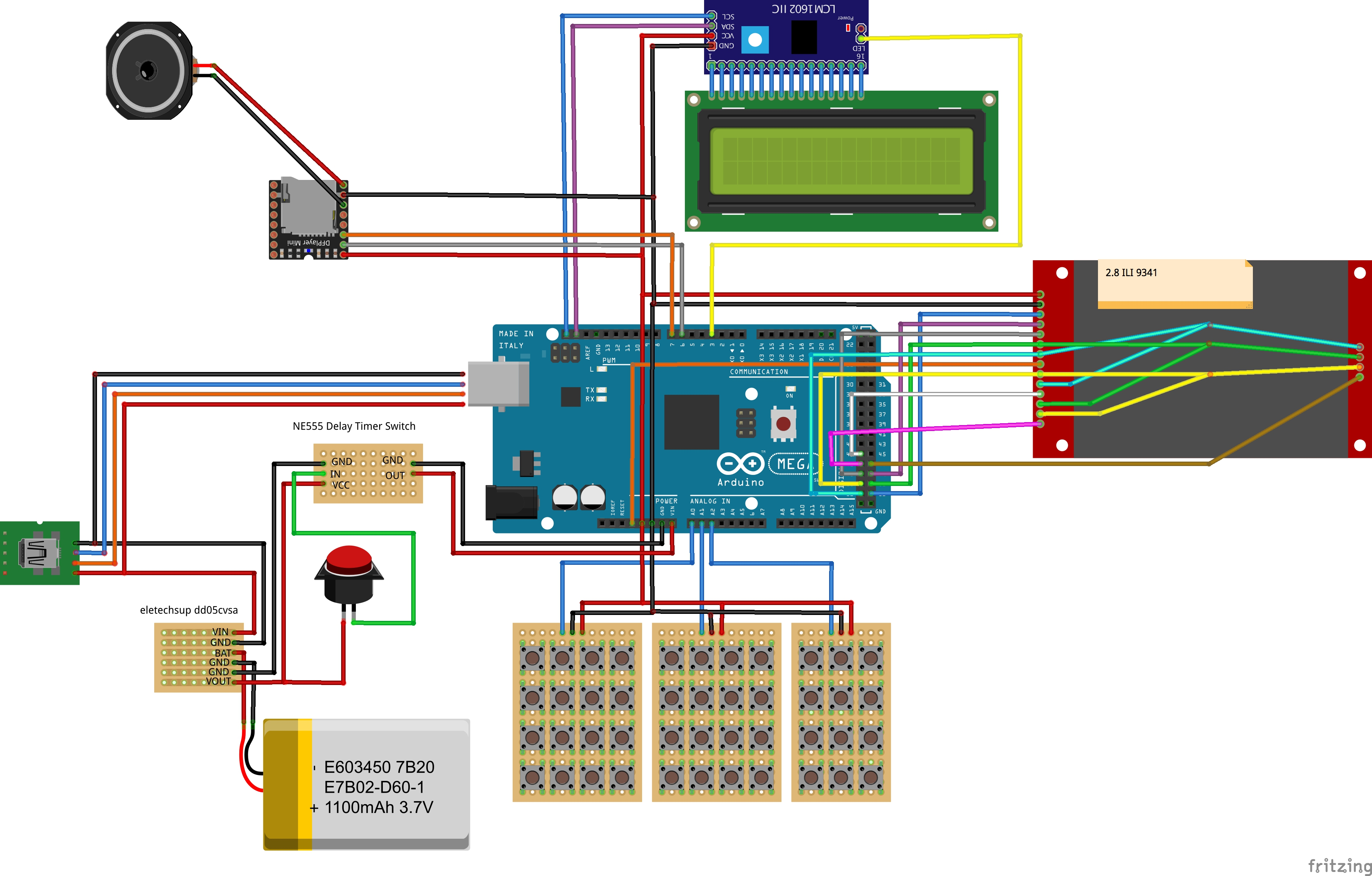lcd__tft__sd_box_xaFPHb6dA1.fzz

#### Raspberry Pi and Arduino Laptop

Project tutorial by Dante Roumega

• 39,275 views
• 103 respects

#### The Talking Alarm Clock

Project tutorial by Abe-Z

• 5,966 views
• 10 respects

#### PLC Training Center

Project tutorial by saifalikabi

• 35,935 views
• 1 comment
• 38 respects

#### Display BMP Pictures from SD Card on TFT LCD Shield

Project tutorial by SurtrTech

• 32,885 views
• 14 respects

#### Reverse Vending Machine (RVM)

Project tutorial by dikshadwivedi1819

• 5,606 views